# Center of line segment

Calculate the distance of the point X [1,3] from the center of the line segment x = 2-6t, y = 1-4t ; t is <0,1>.

Result

d =  4.472

#### Solution:Leave us a comment of example and its solution (i.e. if it is still somewhat unclear...):

Showing 0 comments:Be the first to comment!#### To solve this example are needed these knowledge from mathematics:

Looking for help with calculating arithmetic mean? For Basic calculations in analytic geometry is helpful line slope calculator. From coordinates of two points in the plane it calculate slope, normal and parametric line equation(s), slope, directional angle, direction vector, the length of segment, intersections the coordinate axes etc. Looking for a statistical calculator? Do you want to convert length units? Pythagorean theorem is the base for the right triangle calculator.

## Next similar examples:

1. Equation of circlefind an equation of the circle with indicated properties: a. center at (-3,5), diameter 20. b. center at origin and diameter 16.
2. Segment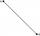Calculate the length of the segment AB, if the coordinates of the end vertices are A[10, -4] and B[5, 5].
3. CablewayCableway has a length of 1800 m. The horizontal distance between the upper and lower cable car station is 1600 m. Calculate how much meters altitude is higher upper station than the base station.
4. Euclid2In right triangle ABC with right angle at C is given side a=27 and height v=12. Calculate the perimeter of the triangle.
5. ABS CNCalculate the absolute value of complex number -15-29i.
6. Median and modusRadka made 50 throws with a dice. The table saw fit individual dice's wall frequency: Wall Number: 1 2 3 4 5 6 frequency: 8 7 5 11 6 13 Calculate the modus and median of the wall numbers that Radka fell.
7. Harmonic and arithmetic means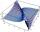The local Utah Department of Child Service office wants to project staffing needs based on current social worker assignments. They have the number of cases per social worker for the following staff: Mary: 25 John: 35 Ted: 15 Lisa: 45 Anna: 20 Calculat
8. Distance problem 2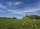A=(x,2x) B=(2x,1) Distance AB=√2, find value of x
9. Variance and average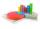Of the 40 values were calculated average mx = 7.5 and variance sx = 2.25. After the control was found to lack the two items of the values of x41 = 3.8 and x42=7. Correct the above characteristics (mx and sx).
10. Hyperbola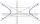Find the equation of hyperbola that passes through the point M [30; 24] and has focal points at F1 [0; 4 sqrt 6], F2 [0; -4 sqrt 6].
11. Circle - AGFind the coordinates of circle and its diameter if its equation is: ?
12. Circle annulusThere are 2 concentric circles in the figure. Chord of larger circle 10 cm long is tangent to the smaller circle. What are does annulus have?
13. Theorem proveWe want to prove the sentense: If the natural number n is divisible by six, then n is divisible by three. From what assumption we started?
14. GP membersThe geometric sequence has 10 members. The last two members are 2 and -1. Which member is -1/16?
15. Vector 7Given vector OA(12,16) and vector OB(4,1). Find vector AB and vector |A|.
16. The ditchDitch with cross section of an isosceles trapezoid with bases 2m 6m are deep 1.5m. How long is the slope of the ditch?
17. 75th percentile (quartille Q3)Find 75th percentile for 30,42,42,46,46,46,50,50,54